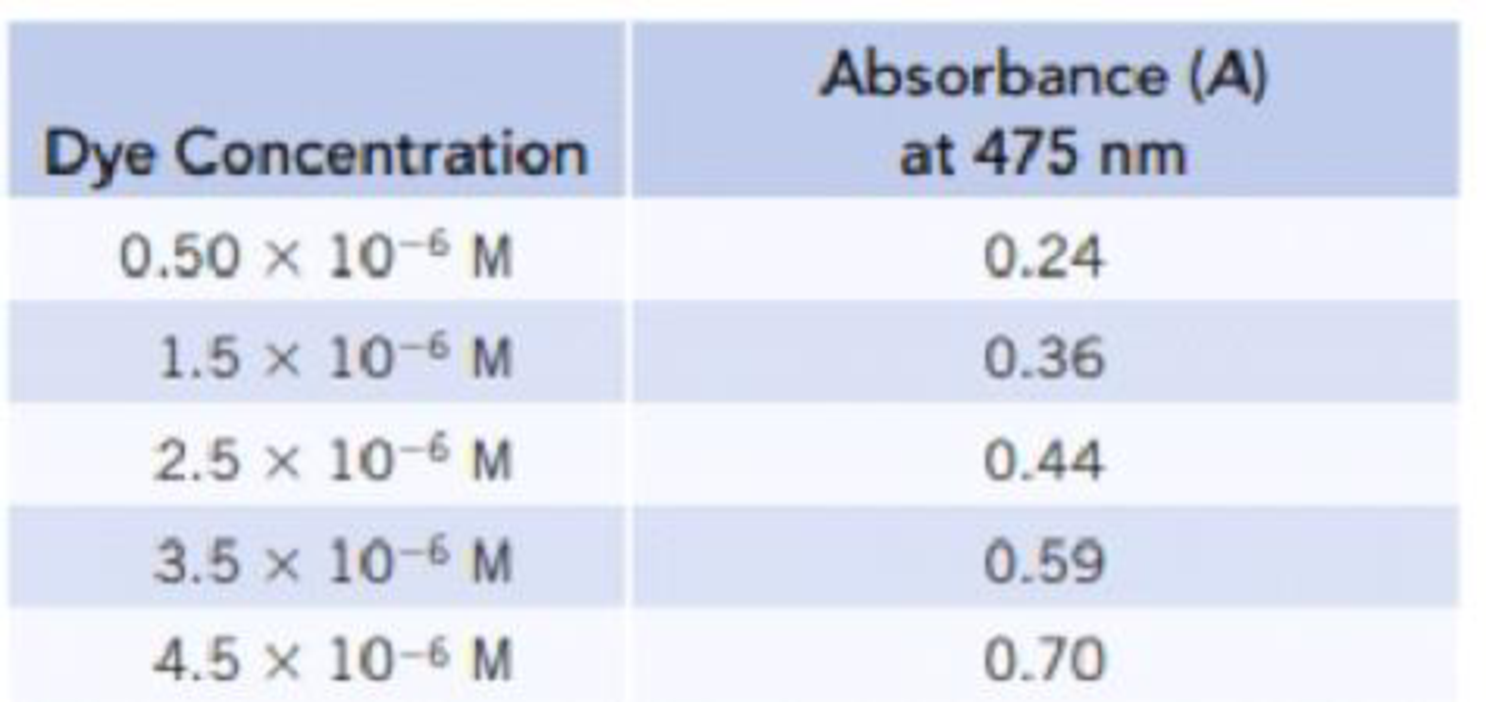Chapter 4, Problem 77PS

Chapter
Section
Textbook Problem

A solution of a dye was analyzed by spectrophotometry, and the following calibration data were collected.(a) Construct a calibration plot, and determine the slope and intercept.(b) What is the dye concentration in a solution with A = 0.52?

(a)

Interpretation Introduction

Interpretation:

By using the given absorbance and concentration values a calibration plot has to be constructed and slope and intercept has to be determined from the calibration plot.

Concept introduction:

• Spectrophotometry is the interaction between light and matter that provides quantitative about solution concentration.
• Absorbance (A): it is the negative logarithm of transmittance of the sample taken. And its value increases as the concentration increases.

Absorbance=-logT=-logPP0

T is the transmittance and it is the ratio of the amount of light transmitted by or passing through the sample (P) relative to the light that initially fell on the sample.

Transmittance(T)=PP0=IntencityoftransmittedlightIntencityofincidentlight

• y=mx+c is a straight line equation where m is the slope and c is the intercept.
• Concentration of the solution can be expressed in many ways; molarity is one of the important parameter to express the concentration of solution. And it is a measure of the concentration of a solute in a solution.
Explanation

A calibration plot is constructed by using the given values of dye concentration with the absorbance (A) as follows,

The equation is,

y=mx+c .....

(b)

Interpretation Introduction

Interpretation:

The concentration of the solution with absorbance (A) 0.52  has to be calculated.

Concept introduction:

• Spectrophotometry is the interaction between light and matter that provides quantitative about solution concentration.
• Absorbance (A): it is the negative logarithm of transmittance of the sample taken. And its value increases as the concentration increases.

Absorbance=-logT=-logPP0

T is the transmittance and it is the ratio of the amount of light transmitted by or passing through the sample (P) relative to the light that initially fell on the sample.

Transmittance(T)=PP0=IntencityoftransmittedlightIntencityofincidentlight

• y=mx+c is a straight line equation where m is the slope and c is the intercept.
• Concentration of the solution can be expressed in many ways, molarity is one of the important parameter to express the concentration of solution.  And it is a measure of the concentration of a solute in a solution.

Still sussing out bartleby?

Check out a sample textbook solution.

See a sample solution

The Solution to Your Study Problems

Bartleby provides explanations to thousands of textbook problems written by our experts, many with advanced degrees!

Get Started

Find more solutions based on key concepts